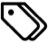## Creating Bandpass Bessel Filter with MATLABMatlab | programming | research | bitbucket

Bessel filters are incredibly useful in numerical analysis, especially for acoustic-type waveforms. This is because analog Bessel filters are characterized by almost constant group delay over any frequency band, and this means that the shape of waves does not change when passed through such a filter.

Well, MATLAB provides some of the building blocks required to create a bandpass analog filter, but does not actually combine the pieces to make a usable filter function.

I created a function for my own research (sourced from pieces I found elsewhere, but it’s been too long—I don’t remember where I found each piece, sorry!), and can be found at my MATLAB repository, specifically, here.

Here’s the documentation that I included with the function:

```besselfilter. Function to implement a bandpass Bessel Filter.

[filtData, b, a] = besselfilter(order,low,high,sampling,data)

Inputs:

- order:      Number of poles in the filter. Scalar numeric value.
Eg.: 4
- low:        Lower frequency bound (Hz). Scalar numeric value.
Eg.: 50000 (= 50kHz)
- high:       Upper frequency bound (Hz). Scalar numeric value.
Eg.: 1000000 (= 1MHz)
- sampling:   Sampling frequency (Hz). Scalar numeric value.
Eg.: 25000000 (= 25MHz)
- data:       Input data. Numeric vector.
Eg.: data vector of size (n x 1)

Output:

- filtData:   Output filtered data. Numeric vector.
Eg.: data vector of size (n x 1)
- b, a:       Transfer function values for the filter. Scalar numeric.
```

## MATLAB repositoryMatlab | programming | research | bitbucket

I’ve been using MATLAB for quite a few years now, using it both for my own research as well for work at VTTI. Well, I decided to share some of the code that I’ve been writing, which may come in handy to others in the same field. I’ve long appreciated the help I’ve received from the larger MATLAB community, and I thought I should start contributing as well. :)

I’ve been using bitbucket as my code repository. I know, GitHub seems to be more popular in the tech and programming community, but by coincidence I happened to learn Mercurial (Hg) first, and Mercurial it has been for me since. Bitbucket supports both Hg and Git, so you may use either based on your preference.

To be sure, these are and will be scripts that I can share, and in some cases may be snippets of code that I think may be useful in implementing certain logic scenarios. They will almost certainly not be pathbreaking new pieces of programming. :) I’ll be glad if you find them useful in any way.

## Matlab: find a string within a cell array of stringsMatlab | research | numerical analysis

I just wanted to jot down a few points about Matlab programming. Specifically, this is about finding a string within another cell array of strings, where the thing I’m really interested in is the index of the cell array where the reference string occurs. For example, if my reference string is `'Gamma'`, and my cell array is `{'Alpha','Beta','Gamma','Delta'}`, then the result of the code should be `3`.

Say,

```cellArray = {'Alpha','Beta','Gamma','Delta','GammaSquared'};
refString = 'Gamma';
```

## Method 1

This method uses the Matlab function `strfind` (link).

```index = strfind(cellArray,refString);
index = find(~cellfun(@isempty,index));
```

Result:

```index =
3   5
```

This method works great if the idea is to find a substring, i.e. in the case where we are looking for all possible matches. It doesn’t work too well, however, if we’re looking for a specific match.

## Method 2

This uses the Matlab function `ismember` (link).

```index = find(ismember(cellArray,refString));
```

Result:

```index =
3
```

Works great if the idea is to find a perfect match. However, let’s also keep tabs on the computation time.

```tic; index = find(ismember(cellArray,refString)); toc;
```

Result:

```Elapsed time is 0.001047 seconds.
```

## Method 3

This uses the Matlab function `strcmp` (link).

```index = find(strcmp(cellArray,refString));
```

Result:

```index =
3
```

Same result as in Method 2, but what about computation time?

```tic; index = find(strcmp(cellArray,refString)); toc;
```

Result:

```Elapsed time is 0.000025 seconds.
```

Turns out Method 3 is more than 41 times faster to execute. So we have a winner!

## Reference

Stack Overflow: How to search for a string in cell array in MATLAB?